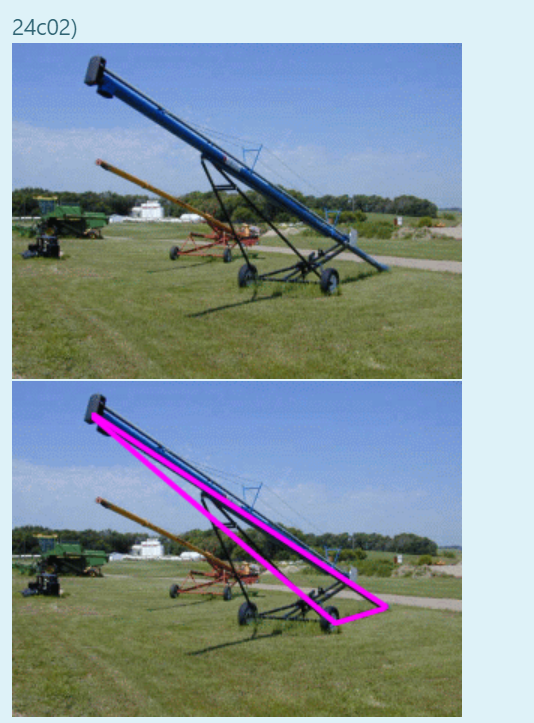# Question Solved1 AnswerCalculate using 4 decimal places for the sin ratio. Here is a picture of a grain auger used to move grain from the ground into a granery. The auger has a crank that is used to raise or lower the top of the auger so that it be used with different sized graneries. This also means that the angles change as well. If the top angle of the auger is 15 degrees, the wheel angle is 113 degrees and the auger is 50 ft in length, then how far is it from the wheels to the base of the auger?Give your answer to 1 decimal place.Put your answer (to 1 decimal place) into answerblank 1 (no spaces) 24c02)Calculate using 4 decimal places for the sin ratio.

Here is a picture of a grain auger used to move grain from the ground into a granery. The auger has a crank that is used to raise or lower the top of the auger so that it be used with different sized graneries. This also means that the angles change as well.
If the top angle of the auger is 15 degrees, the wheel angle is 113 degrees and the auger is 50 ft in length, then how far is it from the wheels to the base of the auger?Give your answer to 1 decimal place.Put your answer (to 1 decimal place) into answerblank 1 (no spaces)Transcribed Image Text: 24c02)
More
Transcribed Image Text: 24c02)